Master ISDD tutorial 2021: Metadynamics simulations with PLUMED

# Aim

The aim of this tutorial is to train users to perform and analyze metadynamics simulations with PLUMED. This tutorial has been prepared by Max Bonomi (adapting a lot of material from other tutorials) for the Master In Silico Drug Design, held at Universite' de Paris on October 26th, 2021.

# Objectives

Once this tutorial is completed users will be able to:

• Write the PLUMED input file to perform metadynamics simulations.
• Calculate the free energy as a function of the metadynamics collective variables.
• Estimate the error in the reconstructed free energies using block analysis.
• Assess the convergence of metadynamics simulations.

# Resources

The for this tutorial contains the following files:

• diala.pdb: a PDB file for alanine dipeptide in vacuo.
• topol.tpr: a GROMACS run (binary) file to perform MD simulations of alanine dipeptide.
• do_block_fes.py: a python script to perform error analysis of metadynamics simulations.

After dowloading the compressed archive to your local machine, you can unpack it using the following command:

tar xvzf master-ISDD-2.tar.gz


Once unpacked, all the files can be found in the master-ISDD-2 directory. To keep things clean, it is recommended to run each exercise in a separate sub-directory that you can create inside master-ISDD-2.

Note
This tutorial has been tested with PLUMED version 2.7.2 and GROMACS version 2019.6.

# Introduction

In the previous tutorial, we have seen that PLUMED can be used to compute collective variables (CVs) on a pre-calculated trajectory. However, PLUMED is most often use to add forces on the CVs during a MD simulation, for example, in order to accelerate sampling. To this aim, we have implemented a variety of possible biases acting on CVs. The complete documentation for all the biasing methods available in PLUMED can be found at the Bias page. In the following we will learn how to use PLUMED to perform and analyze a metadynamics simulation. Here you can find a brief recap of the metadynamics theory.

We will play with a toy system, alanine dipeptide simulated in vacuo using the AMBER99SB-ILDN force field (see Fig. master-ISDD-2-ala-fig). This rather simple molecule is useful to understand data analysis and free-energy methods. This system is a nice example because it presents two metastable states separated by a high free-energy barrier. It is conventional use to characterize the two states in terms of Ramachandran dihedral angles, which are denoted with $$\phi$$ (phi) and $$\psi$$ (psi) in Fig. master-ISDD-2-transition-fig.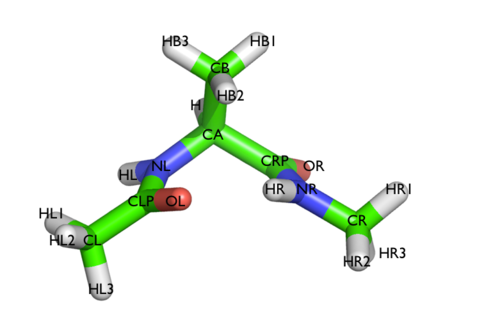The molecule of the day: alanine dipeptide.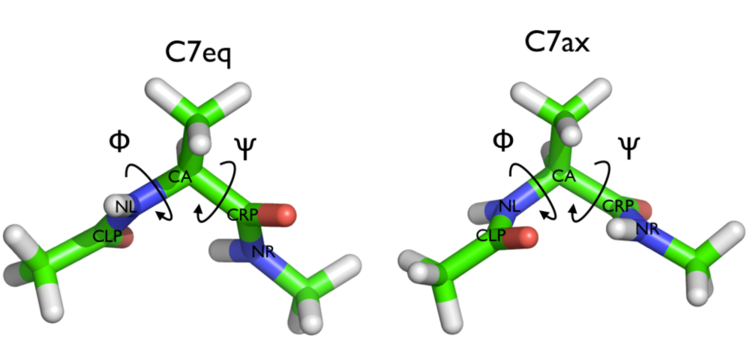Two metastable states of alanine dipeptide are characterized by their Ramachandran dihedral angles.

# Exercises

## Exercise 1: My first metadynamics simulation

In this exercise we will setup and perform a well-tempered metadynamics run using the backbone dihedral $$\phi$$ as collective variable. During the calculation, we will also monitor the behavior of the other backbone dihedral $$\psi$$.

Here you can find a sample plumed.dat file that you can use as a template. Whenever you see an highlighted FILL string, this is a string that you must replace.

Click on the labels of the actions for more information on what each action computes# Activate MOLINFO functionalities
MOLINFO STRUCTUREcompulsory keyword
a file in pdb format containing a reference structure. =diala.pdb
# Compute the backbone dihedral angle phi, defined by atoms C-N-CA-C
# you might want to use MOLINFO shortcuts
phi: TORSION ATOMSthe four atoms involved in the torsional angle =__FILL__
# Compute the backbone dihedral angle psi, defined by atoms N-CA-C-N
# here also you might want to use MOLINFO shortcuts
psi: TORSION ATOMSthe four atoms involved in the torsional angle =__FILL__
# Activate well-tempered metadynamics in phi
metad: __FILL__ ARG could not find this keyword =__FILL__
# Deposit a Gaussian every 500 time steps, with initial height equal to 1.2 kJ/mol
PACE could not find this keyword =500 HEIGHT could not find this keyword =1.2
# The bias factor should be wisely chosen
BIASFACTOR could not find this keyword =__FILL__
# Gaussian width (sigma) should be chosen based on CV fluctuation in unbiased run
SIGMA could not find this keyword =__FILL__
# Gaussians will be written to file and also stored on grid
FILE could not find this keyword =HILLS GRID_MIN could not find this keyword =-pi GRID_MAX could not find this keyword =pi
...
# Print both collective variables on COLVAR file every 10 steps
PRINT ARGthe input for this action is the scalar output from one or more other actions. =__FILL__ FILEthe name of the file on which to output these quantities =COLVAR STRIDEcompulsory keyword ( default=1 )
the frequency with which the quantities of interest should be output =__FILL__


The syntax for the command METAD is simple. The directive is followed by a keyword ARG followed by the labels of the CVs on which the metadynamics bias potential will act. The keyword PACE determines the stride of Gaussian deposition in number of time steps, while the keyword HEIGHT specifies the height of the Gaussian. For each CVs, one has to specify the width of the Gaussian by using the keyword SIGMA. Gaussian will be written to the file indicated by the keyword FILE.

In this example, the bias potential will be stored on a grid, whose boundaries are specified by the keywords GRID_MIN and GRID_MAX. Notice that you can provide either the number of bins for every CV (GRID_BIN) or the desired grid spacing (GRID_SPACING). In case you provide both PLUMED will use the most conservative choice (highest number of bins) for each dimension. In case you do not provide any information about bin size (neither GRID_BIN nor GRID_SPACING) and if Gaussian width is fixed, PLUMED will use 1/5 of the Gaussian width as grid spacing. This default choice should be reasonable for most applications.

Once your plumed.dat file is complete, you can run a 10-ns long metadynamics simulations with the following command:

> gmx mdrun -s topol.tpr -nsteps 5000000 -plumed plumed.dat


During the metadynamics simulation, PLUMED will create two files, named COLVAR and HILLS. The COLVAR file contains all the information specified by the PRINT command, in this case the value of the backbone dihedrals $$\phi$$ and $$\psi$$ every 10 steps of simulation. We can use gnuplot to visualize the behavior of the metadynamics CV $$\phi$$ during the simulation:

gnuplot> p "COLVAR" u 1:2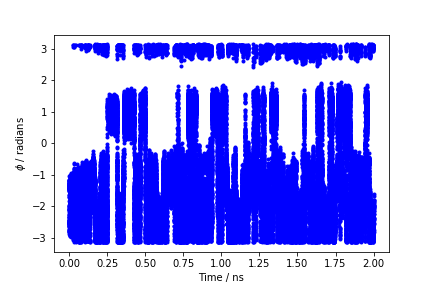Time evolution of the metadynamics CV during the first 2 ns of a metadynamics simulation of alanine dipeptide in vacuum.

By inspecting Figure master-ISDD-2-phi-fig, we can see that the system is initialized in one of the two metastable states of alanine dipeptide. After a while (t=0.1 ns), the system is pushed by the metadynamics bias potential to visit the other local minimum. As the simulation continues, the bias potential fills the underlying free-energy landscape, and the system is able to diffuse in the entire phase space.

The HILLS file contains a list of the Gaussian kernels deposited along the simulation. If we give a look at the header of this file, we can find relevant information about its content:

#! FIELDS time phi sigma_phi height biasf
#! SET multivariate false
#! SET min_phi -pi
#! SET max_phi pi


The line starting with FIELDS tells us what is displayed in the various columns of the HILLS file: the simulation time, the instantaneous value of $$\phi$$, the Gaussian width and height, and the bias factor. We can use the HILLS file to visualize the decrease of the Gaussian height during the simulation, according to the well-tempered recipe: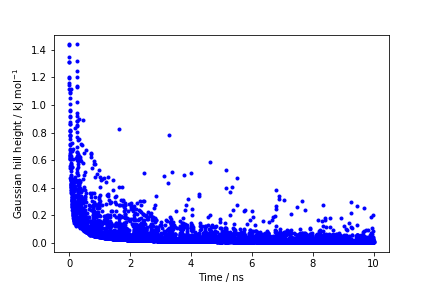Time evolution of the Gaussian height.

If we look carefully at the scale of the y-axis, we will notice that in the beginning the value of the Gaussian height is higher than the initial height specified in the input file, which should be 1.2 kJ/mol. In fact, this column reports the height of the Gaussian scaled by the pre-factor that in well-tempered metadynamics relates the bias potential to the free energy.

Warning
The fact that the Gaussian height is decreasing to zero should not be used as a measure of convergence of your metadynamics simulation!

## Exercise 2: Estimating the free energy as a function of the metadynamics CVs

One can estimate the free energy as a function of the metadynamics CVs directly from the metadynamics bias potential. In order to do so, the utility sum_hills can be used to sum the Gaussian kernels deposited during the simulation and stored in the HILLS file.

To calculate the free energy as a function of $$\phi$$, it is sufficient to use the following command line:

plumed sum_hills --hills HILLS


The command above generates a file called fes.dat in which the free-energy surface as function of $$\phi$$ is calculated on a regular grid. One can modify the default name for the free-energy file, as well as the boundaries and bin size of the grid, by using the following sum_hills options:

--outfile - specify the outputfile for sumhills
--min - the lower bounds for the grid
--max - the upper bounds for the grid
--bin - the number of bins for the grid
--spacing - grid spacing, alternative to the number of bins


The result should look like this: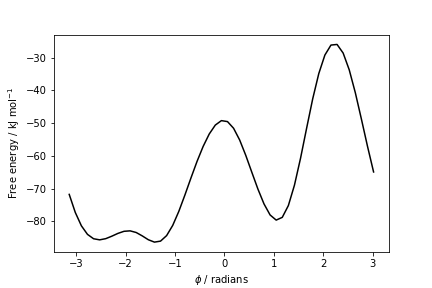Estimate of the free energy as a function of the dihedral phi from a 10ns-long well-tempered metadynamics simulation.

To give a preliminary assessment of the convergence of a metadynamics simulation, one can calculate the estimate of the free energy as a function of simulation time. At convergence, the reconstructed profiles should be similar. The sum_hills option --stride should be used to give an estimate of the free energy every N Gaussian kernels deposited, and the option --mintozero can be used to align the profiles by setting the global minimum to zero. If we use the following command line:

plumed sum_hills --hills HILLS --stride 100 --mintozero


one free energy is calculated every 100 Gaussian kernels deposited, and the global minimum is set to zero in all profiles. The resulting plot should look like the following: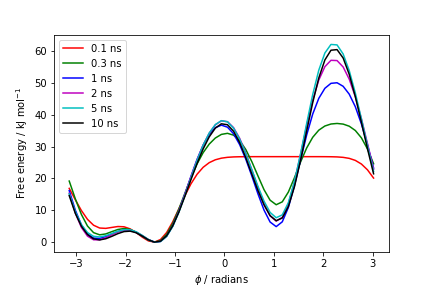Estimates of the free energy as a function of the dihedral phi calculated every 100 Gaussian kernels deposited.

These two qualitative observations:

1. the system is diffusing rapidly in the entire CV space (Figure master-ISDD-2-phi-fig)
2. the estimated free energy does not significantly change as a function of time (Figure master-ISDD-2-metad-phifest-fig)

suggest that the simulation might be converged.

Warning
The two conditions listed above are necessary, but not sufficient to declare convergence. For a quantitative analysis of the convergence of metadynamics simulations, please have a look below at Exercise 4: Estimating the error in free energies using block-analysis.

## Exercise 3: Reweighting (unbiasing) a metadynamics simulation

In the previous exercise we biased $$\phi$$ and computed the free energy as a function of the same variable directly from the metadynamics bias potential using the sum_hills utility. However, in many cases you might decide which variable should be analyzed after having performed a metadynamics simulation. For example, you might want to calculate the free energy as a function of CVs other than those biased during the metadynamics simulation, such as the dihedral $$\psi$$. At variance with standard MD simulations, you cannot simply calculate histograms of other variables directly from your metadynamics trajectory, because the presence of the metadynamics bias potential has altered the statistical weight of each frame. To remove the effect of this bias and thus be able to calculate properties of the system in the unbiased ensemble, you must reweight (unbias) your simulation.

There are multiple ways to calculate the correct statistical weight of each frame in your metadynamics trajectory and thus to reweight your simulation. For example:

1. weights can be calculated by considering the time-dependence of the metadynamics bias potential ;
2. weights can be calculated using the metadynamics bias potential obtained at the end of the simulation and assuming a constant bias during the entire course of the simulation .

In this exercise we will use the second method, which resembles the umbrella-sampling reweighting approach. In order to compute the weights we will use the driver tool.

First of all, you need to prepare a plumed_reweight.dat file that is identical to the one you used for running your metadynamics simulation except for a few modifications. First, you need to add the keyword RESTART=YES to the METAD command. This will make this action behave as if PLUMED was restarting, i.e. PLUMED will read from the HILLS file the Gaussians that have previously been accumulated. Second, you need to set the Gaussian HEIGHT to zero and the PACE to a large number. This will actually avoid adding new Gaussians (and even if they are added they will have zero height). Finally, you need to modify the PRINT statement so that you write every frame and that, in addition to phi and psi, you also write metad.bias. You might also want to change the name of the output file to COLVAR_REWEIGHT.

Click on the labels of the actions for more information on what each action computes# Activate MOLINFO functionalities
MOLINFO STRUCTUREcompulsory keyword
a file in pdb format containing a reference structure. =diala.pdb
__FILL__ # here goes the definitions of the phi and psi CVs
# Activate well-tempered metadynamics in phi
metad: __FILL__ ARG could not find this keyword =__FILL__
# Deposit a Gaussian every 10000000 time steps (never!), with initial height equal to 0.0 kJ/mol
PACE could not find this keyword =10000000 HEIGHT could not find this keyword =0.0 # <- this is the new stuff!
# The bias factor should be wisely chosen
BIASFACTOR could not find this keyword =__FILL__
# Gaussian width (sigma) should be chosen based on CV fluctuation in unbiased run
SIGMA could not find this keyword =__FILL__
# Gaussians will be written to file and also stored on grid
FILE could not find this keyword =HILLS GRID_MIN could not find this keyword =-pi GRID_MAX could not find this keyword =pi
# Say that METAD should be restarting (= reading an existing HILLS file)
RESTART could not find this keyword =YES # <- this is the new stuff!
...
# Print out the values of phi, psi and the metadynamics bias potential
# Make sure you print out the 3 variables in the specified order at every step
PRINT ARGthe input for this action is the scalar output from one or more other actions. =__FILL__ FILEthe name of the file on which to output these quantities =COLVAR_REWEIGHT STRIDEcompulsory keyword ( default=1 )
the frequency with which the quantities of interest should be output =__FILL__ # <- also change this one!


Then run the driver tool using this command:

> plumed driver --mf_xtc traj_comp.xtc --plumed plumed_reweight.dat --kt 2.494339


Notice that you have to specify the value of $$k_BT$$ in energy units. While running your simulation this information was communicated by the MD code.

As a result, PLUMED will produce a new COLVAR_REWEIGHT file with one additional column containing the metadynamics bias potential $$V(s)$$ calculated using all the Gaussians deposited along the entire trajectory. The beginning of the file should look like this:

#! FIELDS time phi psi metad.bias
#! SET min_phi -pi
#! SET max_phi pi
#! SET min_psi -pi
#! SET max_psi pi
0.000000 -1.497988 0.273498 110.625670
1.000000 -1.449714 0.576594 110.873141
2.000000 -1.209587 0.831417 109.742353
3.000000 -1.475975 1.279726 110.752327


You can easily obtain the weight $$w$$ of each frame using the expression $$w\propto\exp\left(\frac{V(s)}{k_BT}\right)$$ (umbrella-sampling-like reweighting). At this point, you can read the COLVAR_REWEIGHT file using, for example, a python script and compute a weighted histogram. Alternatively, if you want PLUMED to do the weighted histograms for you, you can add the following lines at the end of the plumed_reweight.dat file:

Click on the labels of the actions for more information on what each action computes# Use the metadynamics bias as argument
as: REWEIGHT_BIAS ARGcompulsory keyword ( default=*.bias )
the biases that must be taken into account when reweighting =__FILL__
# Calculate histograms of phi and psi dihedrals every 50 steps
# using the weights obtained from the metadynamics bias potentials (umbrella-sampling-like reweighting)
# Look at the manual to understand the parameters of the HISTOGRAM action!
hhphi: HISTOGRAM ARGthe input for this action is the scalar output from one or more other actions. =phi STRIDEcompulsory keyword ( default=1 )
the frequency with which the data should be collected and added to the quantity being
averaged =50 GRID_MINcompulsory keyword
the lower bounds for the grid =-pi GRID_MAXcompulsory keyword
the upper bounds for the grid =pi GRID_BINthe number of bins for the grid =50 BANDWIDTHcompulsory keyword
the bandwidths for kernel density estimation =0.05 LOGWEIGHTSlist of actions that calculates log weights that should be used to weight configurations
when calculating averages =as
hhpsi: HISTOGRAM ARGthe input for this action is the scalar output from one or more other actions. =psi STRIDEcompulsory keyword ( default=1 )
the frequency with which the data should be collected and added to the quantity being
averaged =50 GRID_MINcompulsory keyword
the lower bounds for the grid =-pi GRID_MAXcompulsory keyword
the upper bounds for the grid =pi GRID_BINthe number of bins for the grid =50 BANDWIDTHcompulsory keyword
the bandwidths for kernel density estimation =0.05 LOGWEIGHTSlist of actions that calculates log weights that should be used to weight configurations
when calculating averages =as
# Convert histograms h(s) to free energies F(s) = -kBT * log(h(s))
ffphi: CONVERT_TO_FES GRIDcompulsory keyword
the action that creates the input grid you would like to use =hhphi
ffpsi: CONVERT_TO_FES GRIDcompulsory keyword
the action that creates the input grid you would like to use =hhpsi
# Print out the free energies F(s) to file once the entire trajectory is processed
DUMPGRID GRIDcompulsory keyword
the action that creates the grid you would like to output =ffphi FILEcompulsory keyword ( default=density )
the file on which to write the grid. =ffphi.dat
DUMPGRID GRIDcompulsory keyword
the action that creates the grid you would like to output =ffpsi FILEcompulsory keyword ( default=density )
the file on which to write the grid. =ffpsi.dat


and plot the result using gnuplot:

gnuplot> p "ffphi.dat" u 1:2 w lp
gnuplot> p "ffpsi.dat" u 1:2 w lp


You can now compare the free energies as a function of $$\phi$$ calculated:

1. directly from the metadynamics bias potential using sum_hills as done in Exercise 2: Estimating the free energy as a function of the metadynamics CVs;
2. using the reweighting procedure introduced in this exercise.

The results should be identical (see Fig. master-ISDD-2-fescomp-fig).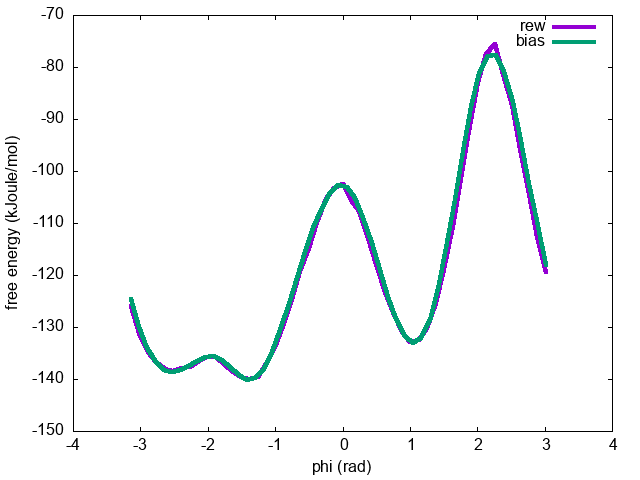Comparison between the free energy as a function of the dihedral phi calculated from the metadynamics bias potential (bias) and by reweighting (rew)

.

## Exercise 4: Estimating the error in free energies using block-analysis

In the previous exercise, we calculated the final bias $$V(s)$$ on the entire metadynamics trajectory and we used this quantity to calculate the correct statistical weight of each frame that we need to reweight the biased simulation. In this exercise, we will see how this information can be used to calculate the error in the reconstructed free energies and assess whether our simulation is converged or not. Let's first calculate the un-biasing weights $$w\propto\exp\left(\frac{V(s)}{k_BT}\right)$$ from the COLVAR_REWEIGHT file obtained at the end of Exercise 3: Reweighting (unbiasing) a metadynamics simulation:

# Find maximum value of bias to avoid numerical errors when calculating the un-biasing weights
bmax=awk 'BEGIN{max=0.}{if($1!="#!" &&$4>max)max=$4}END{print max}' COLVAR_REWEIGHT # Print phi values and un-biasing (un-normalized) weights awk '{if($1!="#!") print $2,exp(($4-bmax)/kbt)}' kbt=2.494339 bmax=$bmax COLVAR_REWEIGHT > phi.weight  If you inspect the phi.weight file, you will see that each line contains the value of the dihedral $$\phi$$ along with the corresponding (un-normalized) weight $$w$$ for each frame of the metadynamics trajectory: 0.907347 0.0400579 0.814296 0.0169656 1.118951 0.0651276 1.040781 0.0714174 1.218571 0.0344903 1.090823 0.0700568 1.130800 0.0622998  At this point we can apply the block-analysis technique (for more info about the theory, have a look at Trieste tutorial: Averaging, histograms and block analysis) to calculate the average free energy across the blocks and the error as a function of block size. For your convenience, you can use the do_block_fes.py python script to read the phi.weight file and produce the desired output. We use a bash loop to test block sizes ranging from 1 to 1000: # Arguments of do_block_fes.py # - input file with CV value and weight for each frame of the trajectory: phi.weight # - number of CVs: 1 # - CV range (min, max): (-3.141593, 3.141593) # - # points in output free energy: 51 # - kBT (kJoule/mol): 2.494339 # - Block size: 1<=i<=1000 (every 10) # for i in seq 1 10 1000; do python3 do_block_fes.py phi.weight 1 -3.141593 3.141593 51 2.494339$i; done


For each value of block size N, you will obtain a separate fes.N.dat file, containing the value of the $$\phi$$ variable on a grid, the average free energy across the blocks with its associated error (in kJ/mol) on each point of the grid:

   -3.141593       23.184653     0.080659
-3.018393       17.264462     0.055181
-2.895194       13.360259     0.047751
-2.771994       10.772696     0.043548
-2.648794        9.403544     0.042022


Finally, we can calculate the average error along each free-energy profile as a function of the block size:

for i in seq 1 10 1000; do a=awk '{tot+=$3}END{print tot/NR}' fes.$i.dat; echo $i$a; done > err.blocks


and visualize it using gnuplot:

gnuplot> p "err.blocks" u 1:2 w lp


As expected, the error increases with the block size until it reaches a plateau in correspondence of a dimension of the block that exceeds the correlation between data points (Fig. master-ISDD-2-block-phi).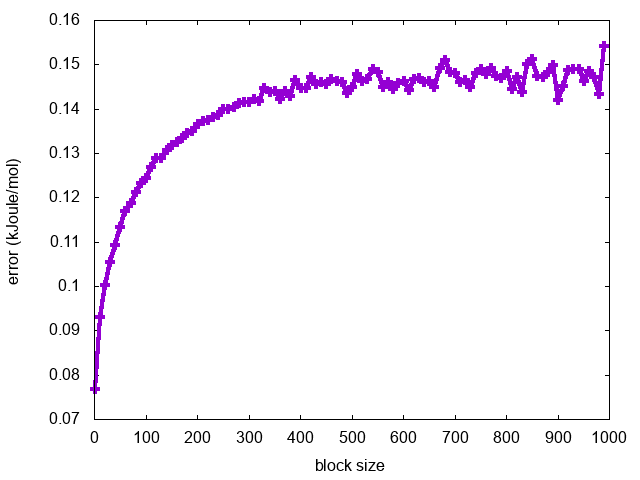Block analysis of a metadynamics simulation using phi as CV

What can we learn from this analysis about the convergence of the metadynamics simulation?

# Conclusions

In summary, in this tutorial you should have learned how to use PLUMED to:

• Setup and run a metadynamics calculation.
• Compute free energies from the metadynamics bias potential using the sum_hills utility.